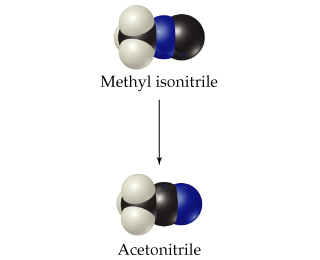# Problem: The activation energy for the isomerization of methyl isonitrile  is 160 kJ/mol.Calculate the fraction of methyl isonitrile molecules that have an energy of 160.0 kJ or greater at 501 K .

###### FREE Expert Solution

Recall that the Arrhenius equation can be written as

k = rate constant

Ea = activation energy (in J/mol)

R = gas constant (8.314 J/mol • K)

T = temperature (in K)

A = Arrhenius constant or frequency factor

85% (68 ratings)###### Problem Details

The activation energy for the isomerization of methyl isonitrileis 160 kJ/mol.

Calculate the fraction of methyl isonitrile molecules that have an energy of 160.0 kJ or greater at 501 K .

Frequently Asked Questions

What scientific concept do you need to know in order to solve this problem?

Our tutors have indicated that to solve this problem you will need to apply the Arrhenius Equation concept. You can view video lessons to learn Arrhenius Equation. Or if you need more Arrhenius Equation practice, you can also practice Arrhenius Equation practice problems.